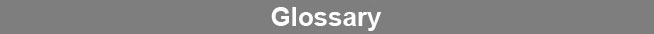Select Page### Weighted Harmonic Mean

The weighted harmonic mean of a set of weighted metric probabilities is the sum of the individual metric weights divided by the sum of the individual weighted reciprocals of the probabilities. A harmonic mean gives a greater effect from very small probabilities than would a weighted arithmetic mean.www.brendan.com